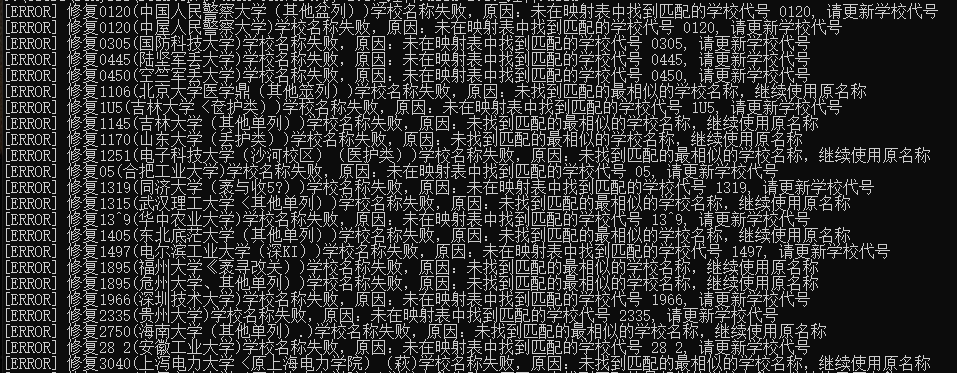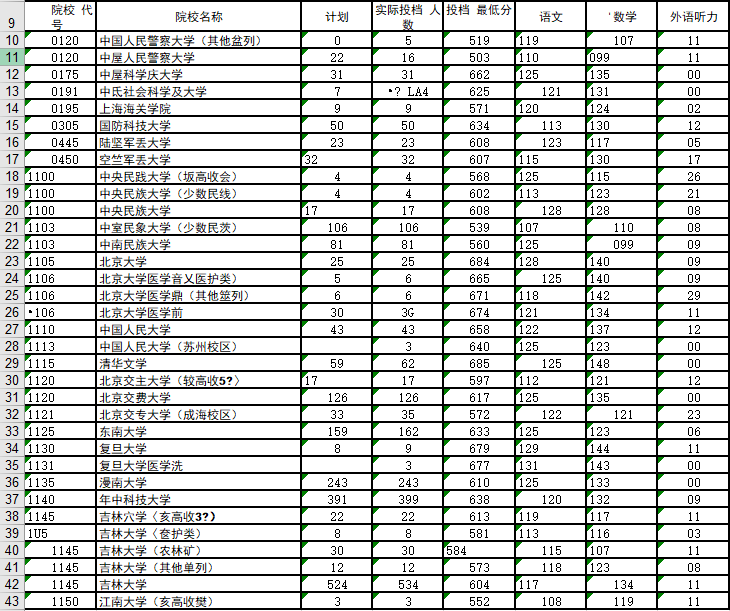# 背景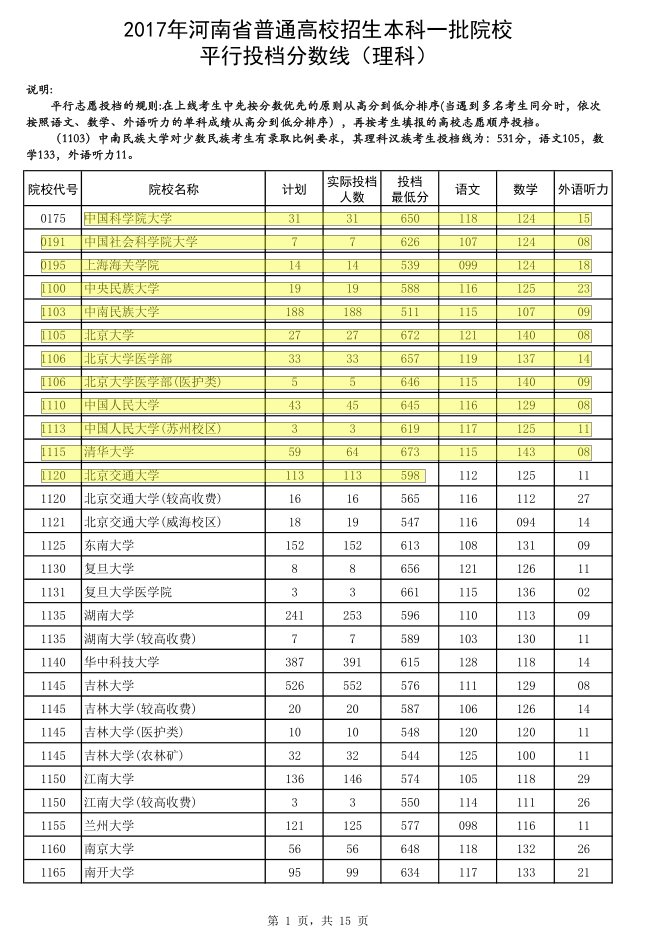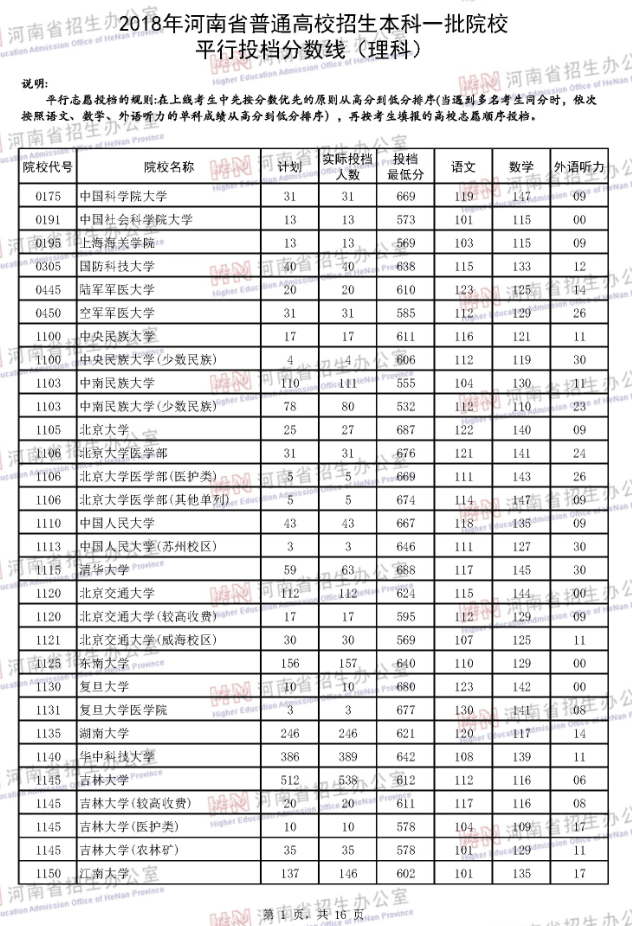# 解决方法

1. 预处理，去掉权限密码校验，以便可以OCR识别。
2. OCR识别，将图片识别为文字。
3. 后处理，修正识别错误的文字。

## 1. 预处理，去掉权限密码校验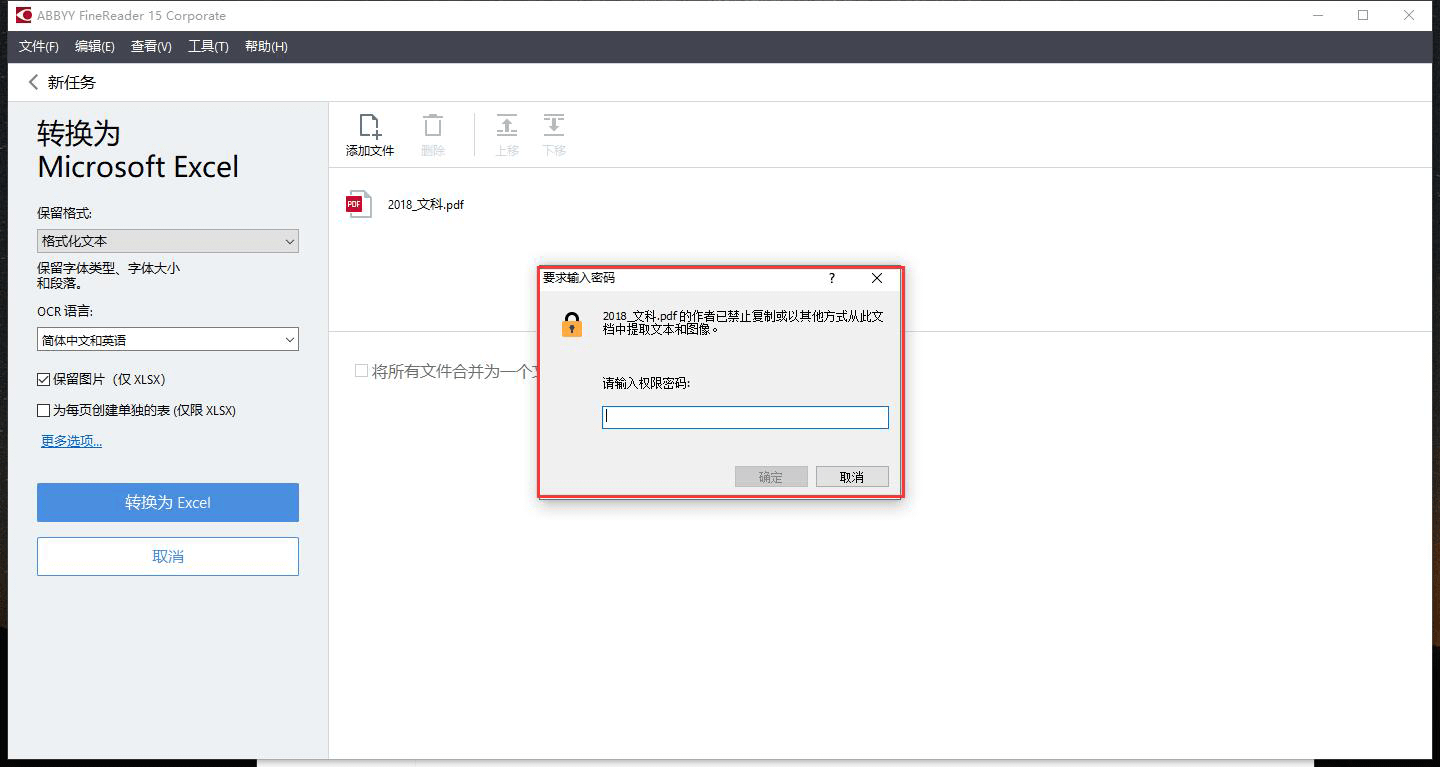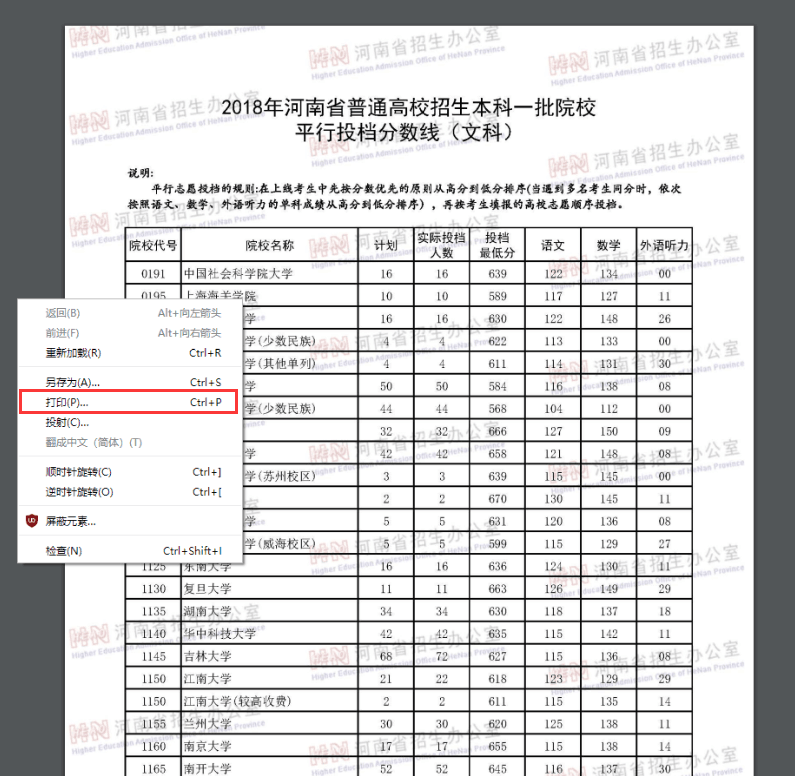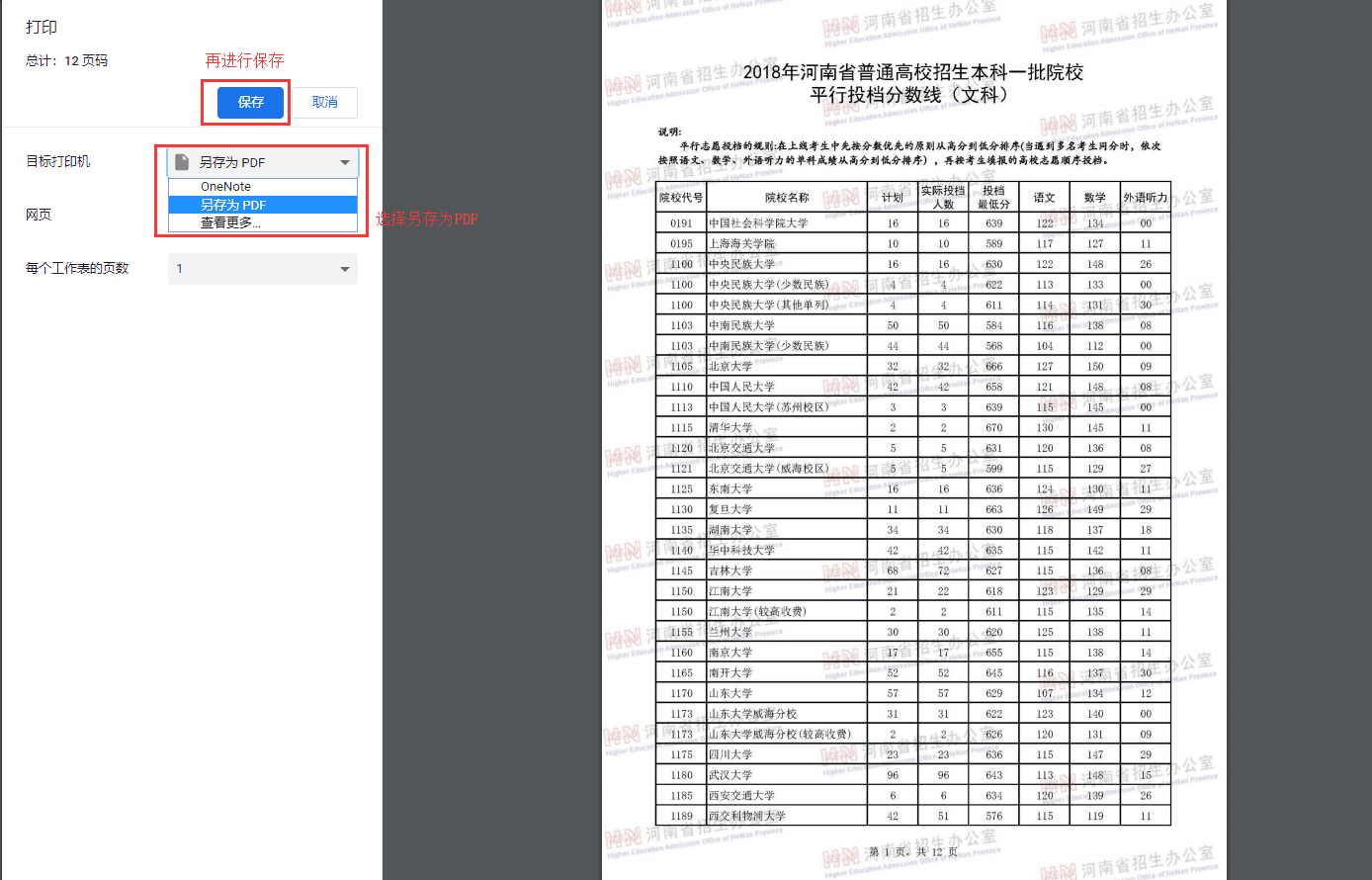## 2. OCR识别，将图片识别为文字

ABBYY FineReader是收费软件，但可以使用试用版本，30天的试用期，基本上够用了，若想长期使用，也可以付费购买或是网上寻找相关的破解版本。

ABBYY FineReader的使用非常简单，这里以最新的15版本为例进行说明。打开软件后，选择转换为Excel格式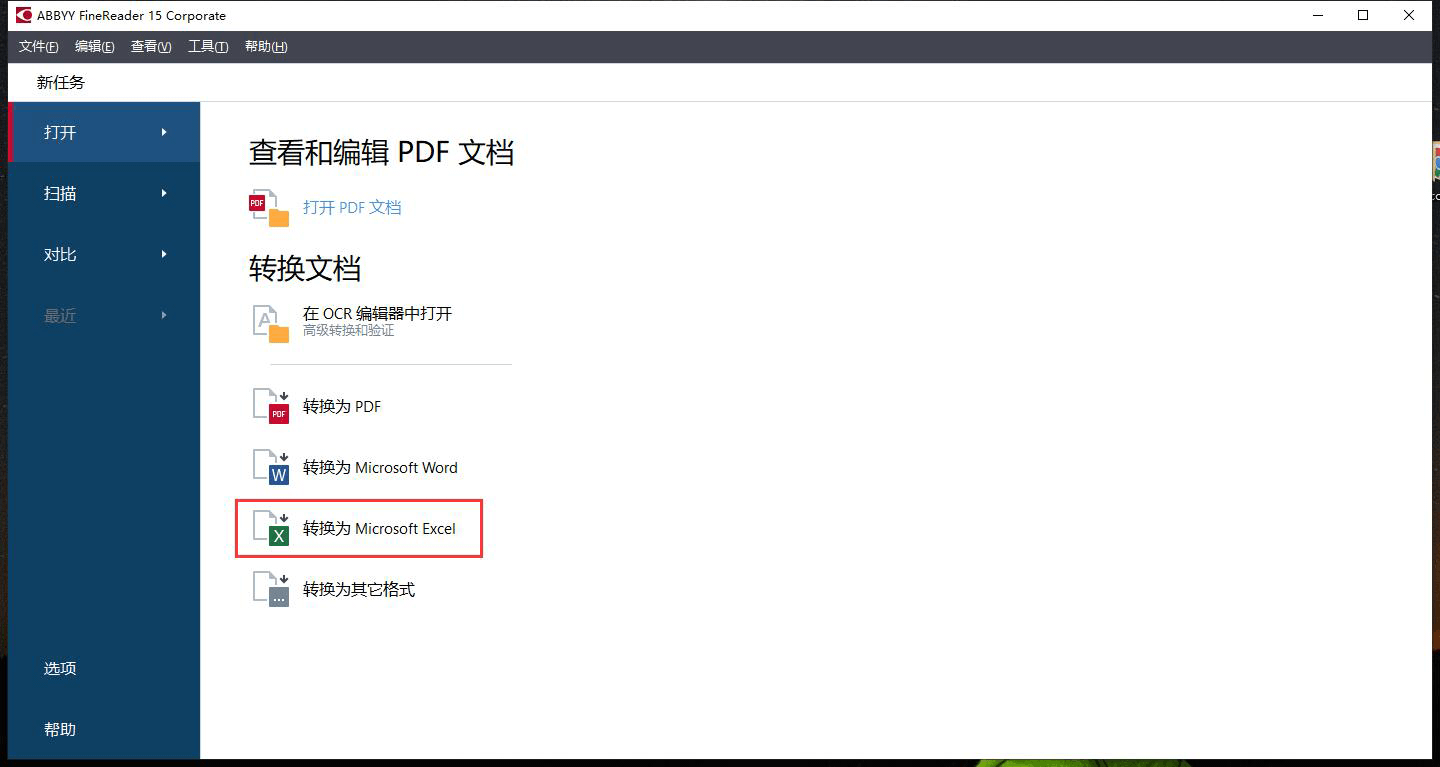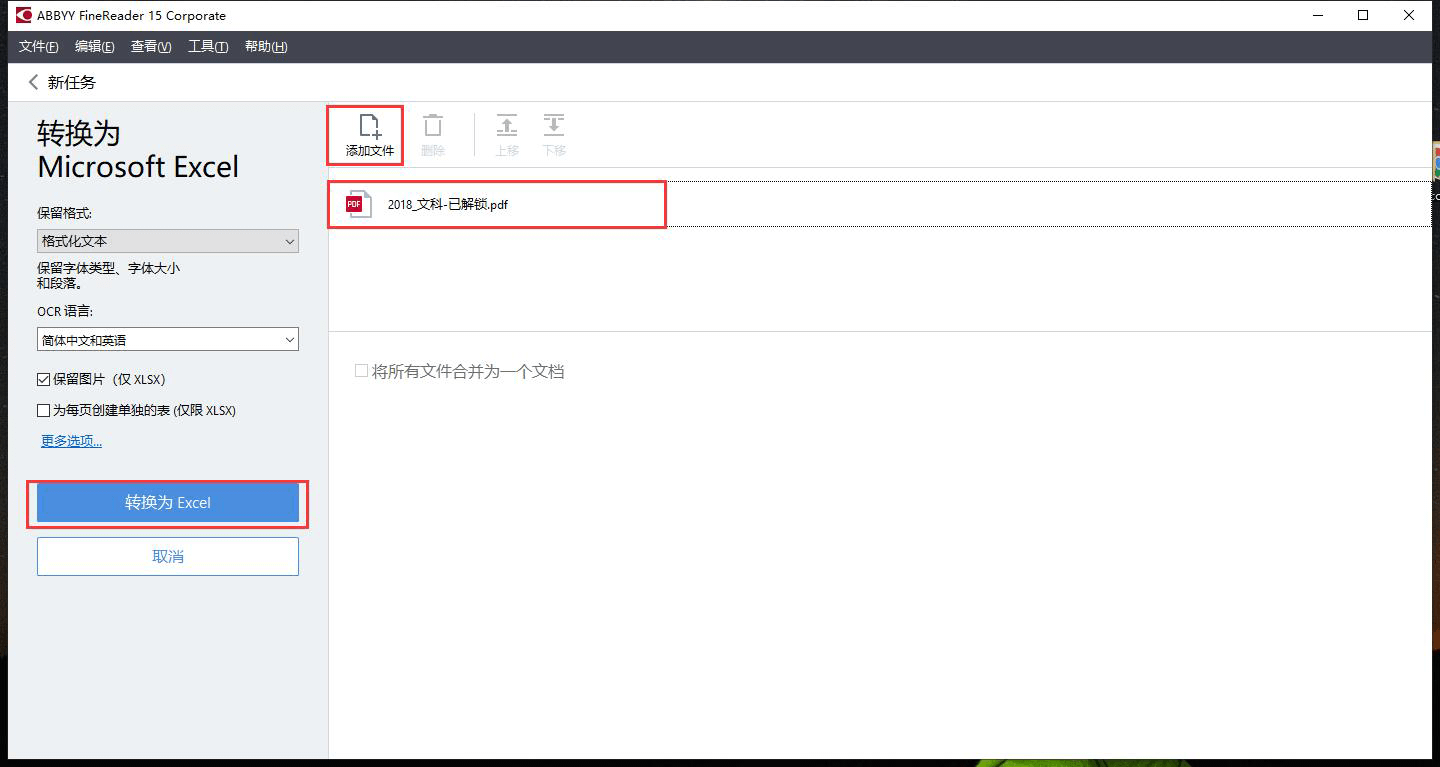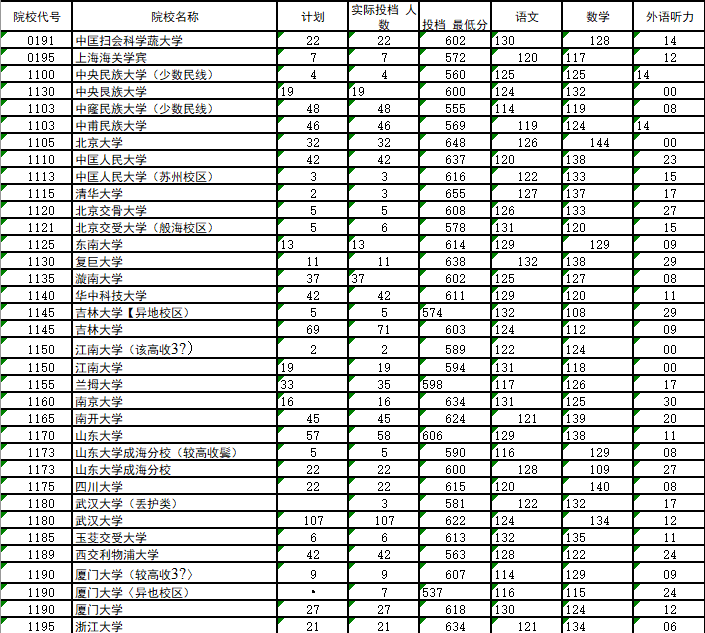## 3. 后处理，修正识别错误的文字

  1 2 3 4 5 6 7 8 9 10 11 12 13 14 15 16 17 18 19 20 21 22 23 24 25 26 27 28 29 30 31 32 33 34 35 36 37 38 39 40 41 42 43 44 45 46 47 48 49 50 51 52 53 54 55 56 57 58 59 60 61 62 63 64 65 66 67 68 69 70 71 72 73 74 75 76 77 78 79 80 81 82 83 84 85 86 87 88 89 90 91 92 93 94 95 96 97 98 99 100 101 102 103 104 105 106 107 108 109 110 111 112 113 114 115 116 117 118 119 120 121 122 123 124 125 126 127 128  # encoding:utf8 import xlrd import xlwt import sys import re def gen_map(base_xls): ''' 用于生成映射表 ''' data = xlrd.open_workbook(base_xls) table = data.sheets() nrows = table.nrows school_map = [] for row in range(nrows): data = table.row_values(row) # 简单过滤 if len([i for i in data if i.isdigit()]) > 5: school_id = data school_name = data school_map.append((school_id, school_name)) return school_map def get_most_similar_name(filter_map, error_school_id, error_school_name): ''' 用于获取最相似的名称，某些相同学校代码下会有多个名称，比如较高学费，医护类等，需要匹配获取最相似的名称 ''' # 相似度至少为1 most_similar_name = (1, None) for m in filter_map: correct_name = m split_error_name = re.split("（|〈|《|、", error_school_name) split_error_count = len(split_error_name) split_correct_name = re.split("（|〈|《|、", correct_name) split_correct_count = len(split_correct_name) if split_error_count == split_correct_count: # 当没有括号时，直接返回正确的名称 if split_correct_count == 1: return correct_name, None compare_error_name = split_error_name[split_error_count - 1].strip() compare_correct_name = split_correct_name[split_correct_count - 1].strip() correct_name_len = len(compare_correct_name) error_name_len = len(compare_error_name) count = correct_name_len if correct_name_len <= error_name_len else error_name_len similar_value = 0 for i in range(count): if compare_correct_name[i] == compare_error_name[i] and compare_correct_name[i] != "）": similar_value += 1 if similar_value >= most_similar_name: most_similar_name = (similar_value, m) if most_similar_name: return most_similar_name, None else: return error_school_name, "未找到匹配的最相似的学校名称，继续使用原名称" def rapir_school_name(school_map, error_data): ''' 修复学校名称 ''' error_school_id = error_data error_school_name = error_data filter_map = [i for i in school_map if i == error_school_id] filter_count = len(filter_map) if filter_count == 0: return error_school_name, "未在映射表中找到匹配的学校代号 %s, 请更新学校代号" %error_school_id else: return get_most_similar_name(filter_map, error_school_id, error_school_name) def rapir(to_rapir_xls, school_map): ''' 遍历有问题的excel行，并根据映射表逐一进行修复 ''' data = xlrd.open_workbook(to_rapir_xls) table = data.sheets() nrows = table.nrows lines = [] error_count = 0 for row in range(nrows): data = table.row_values(row) # 简单过滤，每行至少6个数字 if len([i for i in data if i.isdigit()]) > 5: rapir_name, error_code = rapir_school_name(school_map, data) if error_code: error_count += 1 data.append("×") print("[ERROR] 修复%s(%s)学校名称失败，原因：%s" %(data, data, error_code)) else: data.append("√") data = rapir_name lines.append(data) print("\n[INFO] 共有%d个修复失败项" %error_count) return lines def gen_rapir_xls(name, rapir_data): ''' 生成修复后的excel文件 ''' workbook = xlwt.Workbook() table = workbook.add_sheet("Sheet1") rapir_data.insert(0, ["院校代号", "院校名称", "计划", "实际投档人数", "投档最低分", "语文", "数学", "外语听力", "是否修正"]) for i in range(len(rapir_data)): for j in range(len(rapir_data[i])): table.write(i, j, rapir_data[i][j]) workbook.save(name) print("[INFO]修复后的%s生成成功" %name) def main(): if len(sys.argv) == 3: school_map = gen_map(sys.argv) rapir_data = rapir(sys.argv, school_map) gen_rapir_xls("修复结果.xls", rapir_data) else: print("参数错误，参数格式为: convert <基准excel名称> <待修复的excel名称>") if __name__ == "__main__": main() 

 1 2 3  python convert.py <基准excel名称> <待修复的excel名称> # 一个示例 python convert.py 2017_理科.xlsx 2019_理科.xlsx 

 1  convert.exe 2017_理科.xlsx 2019_理科.xlsx